Home > CAAC > Chapter 5 > Lesson 5.2.3 > Problem5-85

5-85.
1. Find each of the following products by drawing and labeling a generic rectangle or by using the Distributive Property. Homework Help ✎

1. (x + 2)(x + 8)

2. (2m + 30)(m + 20)

3. x(y + 10)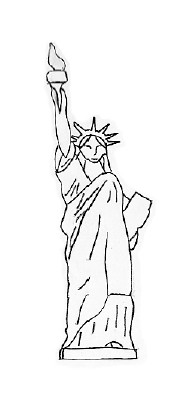4. (2x + 3)(3x + 4)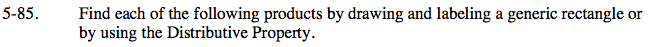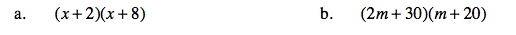Create a generic rectangle.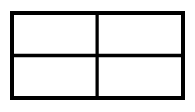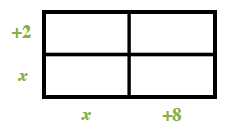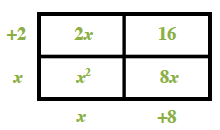Put the products on the outside.

Multiply the expressions.

Write the areas as a sum.
x² + 2x + 8x + 16

(x + 2)(x + 8) = x² + 10x + 16

Follow the steps in part (a).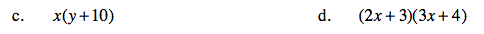Use the Distributive Property.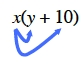x(y + 10) = xy + 10x

Follow the steps in part (a).

(2x + 3)(3x + 4) = 6x² + 17x + 12